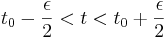# 7.1: Dirac delta (impulse) function

•• Contributed by Peter Woolf et al.
• Assistant Professor (Chemical Engineering) at University of Michigan

## 1.1 Introduction

The Dirac delta function δ(tt0) is a mathematical idealization of an impulse or a very fast burst of substance at t = t0. (Here we are considering time but the delta function can involve any variable.) The delta function is properly defined through a limiting process. One such definition is as a thin, tall rectangle, of width ε:

$\delta\left(t-t_{0}\right)=\frac{1}{\epsilon}$

for

$t_{0}-\frac{\epsilon}{2}<t<t_{0}+\frac{\epsilon}{2}$

and zero otherwise, in the limit that $$\epsilon \rightarrow 0$$.

Then, we have

$\int_{a}^{b} \delta\left(t-t_{0}\right) d t=\lim _{\epsilon \rightarrow 0}\left[\epsilon \cdot\left(\frac{1}{\epsilon}\right)\right]=1$

as long as a < t0 < b. When t0 is outside the range of (a,b), then the integral is zero.

Likewise, for any function $$f(t)$$ that is continuous and differentiable (analytic) at t0,where the quantity in the square brackets above is just the average value of f(t) in the interval. Thus, when, it becomes just the value at t0.

## 1.2 Examples## 1.3 Delta function at the initial time

Note: if one of the limits of the integral coincides exactly with $$t_0$$, then the result is usually cut in half:

$\int_{t_{0}}^{b} \delta\left(t-t_{0}\right) f(t) d t=f\left(t_{0}\right) / 2$

for b > t0. For example:

$\int_{2}^{\infty} \delta(t-2) t^{2} d t=(1 / 2) 2^{2}=2$

However, when we think of an impulse to a system at the initial time t0, then we really consider that the entire delta function is added to the system - that is, the actual time is an infinitesimal amount beyond $$t_0$$; that is, $$t=t_{0}^{+}$$. In that case

$\int_{t_{0}}^{b} \delta\left(t-t_{0}^{+}\right) f(t) d t=f\left(t_{0}\right)$

## 1.4 Definition in terms of a Gaussian

Another equivalent definition of the delta functions are as a Gaussian function:

$\delta\left(t-t_{0}\right)=\lim _{\sigma \rightarrow 0} \frac{1}{\sigma \sqrt{2 \pi}} e^{-\frac{\left(t-t_{0}\right)^{2}}{2 \sigma^{2}}}$

All the properties work out to be the same where $$a=\sqrt{2} \sigma$$ is used.

## 1.5 Physical applications

In Control, the delta function is used an idealization of a very fast perturbation to the system. For example, if you dump a bucket of water into a tank, then the "flow rate" is essentially a delta function - a very highly peaked function, but with a net integral (the total amount of water in the bucket).

In mechanics, and example of the delta function is the force when hitting an object by a hammer. Say you hit a steel ball with a hammer. It moves with a certain velocity representing the total momentum transferred by the hammer. Rather than talk about the force x time (the net momentum transfer), one talks about an "impulse" which is the net momentum transferred in an infinitesimally short amount of time.

## 1.6 Relation to the step function

The step function, $$Θ(t − t_0)$$, is the integral of the delta function or alternatively, the delta function is the derivative of the theta function, where $$Θ(t − t_0)$$ is defined at 1 for $$t > t_0$$ and 0 for $$t < t_0$$:.

Here, the smooth or gaussian definition of the delta function corresponds to a smooth representation of the Θ function as the integral of a gaussian or equivalently, the error function.